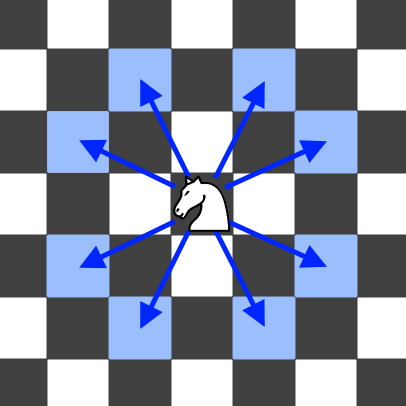704. Knight Probability in Chessboard

0

Medium

Given an n x n chessboard, a knight starts at a specific cell (row, column) and aims to make exactly k moves. The chessboard is 0-indexed, with the top-left cell being (0, 0) and the bottom-right cell being (n - 1, n - 1). A knight can make eight possible moves, as shown in the diagram below. Each move consists of two cells in a cardinal direction, followed by one cell in an orthogonal direction.At each move, the knight randomly selects one of the eight possible moves (even if it would go off the chessboard) and moves accordingly. The knight continues moving until it has made exactly k moves or has moved off the chessboard. Calculate the probability that the knight remains on the board after it has stopped moving.

Input Format

The first line contains the value of n. The second line contains the value of k. The third line contains the value of row. The fourth line contains the value of column.

Output Format

Print the probability that the knight remains on the board after it has stopped moving as an integer.

Example

Input

3 2 0 0

Output

0.06250

Constraints

1 <= n <= 25
0 <= k <= 100
0 <= row, column <= n - 1